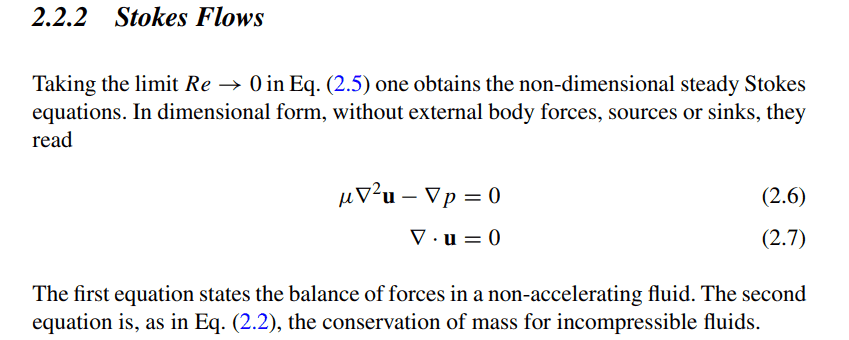# External forces on Stokes flow

Est120
Homework Statement:
find the appropiate Navier-Stokes equation in the low Re number limit
Relevant Equations:
Navier Stokes equations
in the limit as Re→0 , we can neglect the material derivate of v ( Dv/Dt =0 ) but why in books they always make the gravity effects equal to 0?
i can't understand and no one really explains this stuffThe density is being treated as constant, so the gravational force per unit mass is $\mathbf{g} = -\nabla\left(-\int\mathbf{g} \cdot d\mathbf{x}\right)$.
You can therefore absorb it into the pressure term. It makes no difference to the mathematics if you work with $p$ or $p' = p - \int\mathbf{g} \cdot d\mathbf{x}$.
The density is being treated as constant, so the gravational force per unit mass is $\mathbf{g} = -\nabla\left(-\int\mathbf{g} \cdot d\mathbf{x}\right)$.
You can therefore absorb it into the pressure term. It makes no difference to the mathematics if you work with $p$ or $p' = p - \int\mathbf{g} \cdot d\mathbf{x}$.